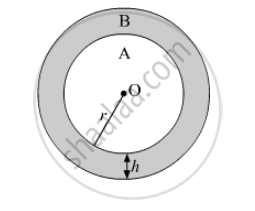Advertisement Remove all ads

# Prove that the area of a circular path of uniform width h surrounding a circular region of radius r is π h ( 2 r + h ) - Mathematics

Sum

Prove that the area of a circular path of uniform width h surrounding a circular region of radius r is pih(2r+h)

Advertisement Remove all ads

#### SolutionThe width of the circular path = h
Let the inner circle be region A and the outer circle be region B
Radius of region A = r
Radius of region B = r + h
Area of the circular path = Area of region B − Area of region A

= pi(r+h)^2-pir^2

= pi(r^2+h^2+2rh-r^2)

= pih(h+2r)

Hence Proved

Is there an error in this question or solution?
Advertisement Remove all ads

#### APPEARS IN

RD Sharma Class 10 Maths
Chapter 13 Areas Related to Circles
Exercise 13.1 | Q 31 | Page 13
Advertisement Remove all ads

#### Video TutorialsVIEW ALL 

Advertisement Remove all ads
Share
Notifications

View all notifications

Forgot password?
Course